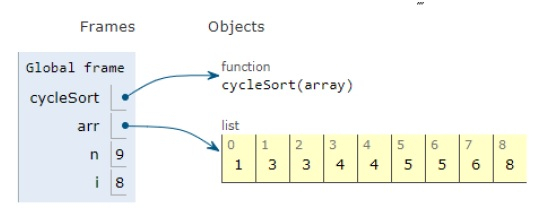# Python Program for Cycle Sort

Problem statement − We are given an array, we need to sort it using the concept of cycle sort.

It is an in-place algorithm and swapping takes place by the formation of cycles.

Now let’s observe the solution in the implementation below −

## Example

Live Demo

def cycleSort(array):
writes = 0
# cycles to be rotated
for cycleStart in range(0, len(array) - 1):
item = array[cycleStart]
#position to place the item
pos = cycleStart
for i in range(cycleStart + 1, len(array)):
if array[i] < item:
pos += 1
# if item exits, it is not a cycle
if pos == cycleStart:
continue
# Otherwise, place the item
while item == array[pos]:
pos += 1
array[pos], item = item, array[pos]
writes += 1
# rotation continued
while pos != cycleStart:
# Find a position to place the item
pos = cycleStart
for i in range(cycleStart + 1, len(array)):
if array[i] < item:
pos += 1
# place the item
while item == array[pos]:
pos += 1
array[pos], item = item, array[pos]
writes += 1
return writes
# main
arr = [1,5,3,4,8,6,3,4,5]
n = len(arr)
cycleSort(arr)
print("Sorted array is : ")
for i in range(0, n) :
print(arr[i], end = " ")

## Output

Sorted array is :
1 3 3 4 4 5 5 6 8All the variables are declared in the local scope and their references are seen in the figure above.

## Conclusion

In this article, we have learned about how we can make a Python Program for Cycle Sort

Updated on: 20-Dec-2019

152 Views